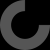MakerBot Print is our newest print-prepration software, which supports native CAD files and STL assemblies,
allows you to interact with all your printers via the Cloud, and many other exciting new features.

Raspberry Pi Case with OLED SSD1306

Oct 28, 2017

Thing Apps Enabled

I seriously love this design and would like to know exactly what OLED it has been designed for. I've also noticed that the outer wall above the OLED is too thin. Thanks for the great design!

thanks for the comment, the OLED here is just one of those from Ebay/China. This is a link to one

search for 128x32 OLED and I2C makes an easy way for it to be driven from a Pi

Havent had an issue with the wall, I was using CURA as a slicer, I have Slic3r now and will try with that in case its a geometry thing.

Thanks for the reply. I can't wait to get the OLEDs installed. I've posted a second make highlighting the 'thin' wall. I really like your design. Thanks!

Looks like Slic3r doesnt like that area, I've put a temp shim in an upload and will redo it and fire off a test print, thanks for the heads up

HI
first you'll need to install PiHole and the status script also uses the Luma core package that will also need to be installed. I find this the easiest to use and also it handles the screen rotation.

#!/usr/bin/env python
# -*- coding: utf-8 -*-
# PYTHON_ARGCOMPLETE_OK

"""
Clock Ported from: https://gist.github.com/TheRayTracer/dd12c498e3ecb9b8b47f#file-clock-py
"""

import math
import time
import datetime
import subprocess
import json
import requests
import RPi.GPIO as GPIO

GPIO.setmode(GPIO.BCM)
GPIO.setwarnings(False)
GPIO.setup(23,GPIO.OUT)
GPIO.output(23,GPIO.HIGH)

## enter your IP address here of your pihole installation

from luma.core.render import canvas
from luma.core.interface.serial import i2c
from luma.oled.device import ssd1306

serial = i2c(port=1, address=0x3C)
device = ssd1306(serial,width=128, height=32, rotate=3)

def posn(angle, arm_length):
dx = int(math.cos(math.radians(angle)) * arm_length)
dy = int(math.sin(math.radians(angle)) * arm_length)
return (dx, dy)

def main():
today_last_time = "Unknown"
while True:

now = datetime.datetime.now()
today_date = now.strftime("%d/%m")
today_time = now.strftime("%H:%M")
GPIO.output(23,GPIO.LOW)

if today_time != today_last_time:
# Pi Hole data!
try:
r = requests.get(api_url)
DNSQUERIES = data['dns_queries_today']
CLIENTS = data['unique_clients']
except:
time.sleep(.2)
continue

GPIO.output(23,GPIO.HIGH)
today_last_time = today_time
with canvas(device) as draw:
now = datetime.datetime.now()
today_date = now.strftime("%d/%m")

margin = 0

cx = 16
cy = 15
ecy = 30

left = cx - cy
right = cx + cy

hrs_angle = 270 + (30 * (now.hour + (now.minute / 60.0)))
hrs = posn(hrs_angle, cy - margin - 7)

min_angle = 270 + (6 * now.minute)
mins = posn(min_angle, cy - margin - 2)

sec_angle = 270 + (6 * now.second)
secs = posn(sec_angle, cy - margin - 2)

draw.ellipse((left + margin, margin, right - margin, ecy), outline="white")

draw.line((cx, cy, cx + hrs, cy + hrs), fill="white")
draw.line((cx, cy, cx + mins, cy + mins), fill="white")
#draw.line((cx, cy, cx + secs, cy + secs), fill="red")
draw.ellipse((cx - 2, cy - 2, cx + 2, cy + 2), fill="white", outline="white")

draw.text((( margin), ecy + 8), today_time, fill="yellow")
draw.text((( margin), ecy +16), today_date, fill="yellow")

bstart = 30
draw.text((margin,ecy + bstart),"Ads"           , fill="white")
draw.text((margin,ecy + bstart+8),str(ADSBLOCKED)       , fill="white")

cstart= 52
draw.text((margin,ecy + cstart),"Users"                 , fill="white")
draw.text((margin,ecy + cstart+8),str(CLIENTS)  , fill="white")

dstart = 74
draw.text((margin,ecy + dstart),chr(35)+"DNS"           , fill="white")
draw.text((margin,ecy + dstart+8), str(DNSQUERIES)      , fill="white")

time.sleep(0.1)
GPIO.output(23,GPIO.HIGH)

if __name__ == "__main__":
try:
main()
except KeyboardInterrupt:
pass